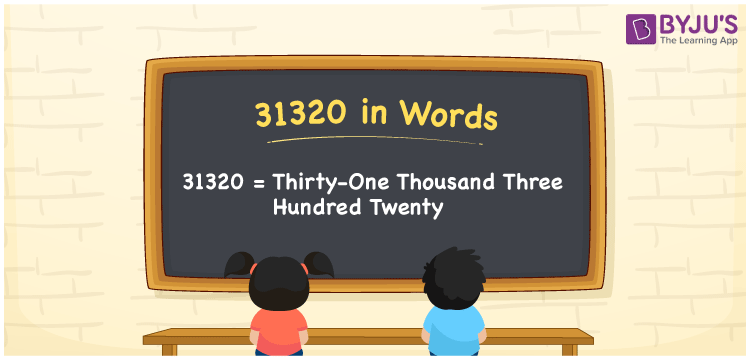# 31320 in words

31320 in words is written as Thirty One Thousand Three Hundred and Twenty. In 31320, the first 3 has a place value of ten thousand, 1 has a place value of thousand, the next 3 has the place value of hundred and 2 is in the place value of ten. The article on Place Value gives more information. The number 31320 is used in expressions that relate to money, distance, length, social media views, and many more. For example, “A famous cricket player’s career has a total of Thirty One Thousand Three Hundred and Twenty runs to his credit”

 31320 in words Thirty One Thousand Three Hundred and Twenty Thirty One Thousand Three Hundred and Twenty in Numbers 31320

## 31320 in English Words## How to Write 31320 in Words?

We can convert 31320 to words using a place value chart. This can be done as follows. The number 31320 has 5 digits, so let’s make a chart that shows the place value up to 5 digits.

 Ten thousand Thousands Hundreds Tens Ones 3 1 3 2 0

Thus, we can write the expanded form as:

3 × Ten thousand + 1 × Thousand + 3 × Hundred + 2 × Ten + 0 × One

= 3 × 10000 + 1 × 1000 + 3 × 100 + 2 × 10 + 0 × 1

= 31320.

= Thirty One Thousand Three Hundred and Twenty.

31320 is the natural number that is succeeded by 31319 and preceded by 31321.

31320 in words – Thirty One Thousand Three Hundred and Twenty.

Is 31320 an odd number? – No.

Is 31320 an even number? – Yes.

Is 31320 a perfect square number? – No.

Is 31320 a perfect cube number? – No.

Is 31320 a prime number? – No.

Is 31320 a composite number? – Yes.

## Solved Example

1. Write the number 31320 in expanded form

Solution: 3 x 10000 + 1 x 1000 + 3 x 100 + 2 x 10 + 0 x 1

Or Just 3 x 10000 + 1 x 1000 + 3 x 100 + 2 x 10

We can write 31320 = 30000 + 1000 + 300 + 20 + 0

= 3 x 10000 + 1 x 1000 + 3 x 100 + 2 x 10 + 0 x 1.

## Frequently Asked Questions on 31320 in words

Q1

### How to write the number 31320 in words?

31320 in words is written as Thirty One Thousand Three Hundred and Twenty.
Q2

### Is 31320 divisible by 3?

Yes. 31320 is divisible by 3.
Q3

### Is 31320 a prime number?

No. 31320 is not a prime number.